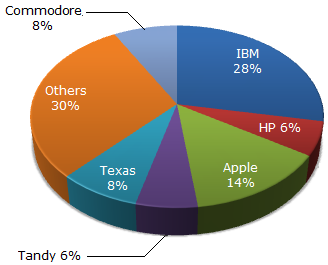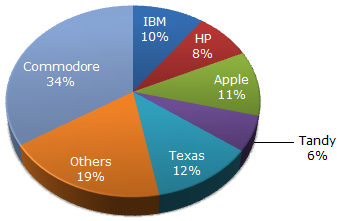# Data Interpretation - Pie Charts - Discussion

The pie chart shows the distribution of New York market share by value of different computer companies in 2005.The pie chart shows the distribution of New York market share by volume of different computer companies in 2005.

Number of units sold in 2005 in New York = 1,500

Value of units sold in 2005 in New York = US \$1,650,000.3.

In 2005, the average unit sale price of an IBM PC was approximately (in US\$)

 [A]. 3180 [B]. 2800 [C]. 393 [D]. 3080

Explanation:

IBM accounts for 28% of the share by value and 10% of the share by volume.

28% of 1650000 = 28 x 1650000/100 = 462000

10% of 1500 = 10 x 1500/100 = 150

Therefore, average unit sale price = 462000/150 = 3080.

 Sandeep Wasnik said: (May 20, 2017) Someone please explain in detail.

 Gnaneswari said: (Jul 22, 2018) Can you explain in detail?

 Paul said: (Aug 23, 2018) It should be 393.

 Niranjan said: (Sep 1, 2018) Calculation is right. Because only part of IBM Pc which in VALUE and VOLUME (\$ per unit) for calculating of average of IBM PC.